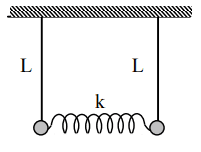# Coupled oscillators -- period of normal modes

Homework Statement:
I have to determine the periods of the normal modes.

I have 2 mass ##m_1 = m_2##, l = 1.2m and if I hold vertically one of the mass the period of the other mass is 1.5s.
Relevant Equations:
F = ma

##m\ddot x = -\frac{mgx_1}{l} -k(x_1 - x_2)##
##m\ddot x_2 = -\frac{mgx_2}{l} +k(x_1 - x_2)##
Hi,

I know there's are 2 normal modes because the system has 2 mass. I did the newton's law for both mass.

##m\ddot x_1 = -\frac{mgx_1}{l} -k(x_1 - x_2)## (1)
##m\ddot x_2 = -\frac{mgx_2}{l} +k(x_1 - x_2)## (2)

In the pendulum mode ##x_1 = x_2## and in the breathing mode ##x_1 = -x_2##

I get the pendulum and breathing mode by adding equation 1 and 2 and subtracting 1 and 2 then I replace ##x_1-x_2## by q2 and ##x_1+x_2## by q1.
Finally I have ##\omega_p = \sqrt{\frac{g}{l}}## and ##\omega_b = \sqrt{\frac{g}{l} + \frac{2k}{m}}##
It's quite easy to get the period for ##\omega_p##. However, I'm not sure how to find the period for ##\omega_b##, since I don't have k and m.Last edited by a moderator:

haruspex
Homework Helper
Gold Member
2020 Award
You will need to supply a diagram or a full description of the physical arrangement. In particular, I am confused regarding whether these x displacements are horizontal or vertical.

You will need to supply a diagram or a full description of the physical arrangement. In particular, I am confused regarding whether these x displacements are horizontal or vertical.

haruspex
Homework Helper
Gold Member
2020 Award
Doesn't this tell you k/m?
"if I hold vertically one of the mass the period of the other mass is 1.5s."

•EpselonZero
Doesn't this tell you k/m?
"if I hold vertically one of the mass the period of the other mass is 1.5s."

I need to find the period for the normal modes. I don't see the link between 1.5s and the normal modes.

haruspex
Homework Helper
Gold Member
2020 Award
I need to find the period for the normal modes. I don't see the link between 1.5s and the normal modes.
You wrote that what was blocking you was that you did not know k/m.
What wouid the period be if you suspended one of the masses from the other and let it oscillate vertically?

I see...

Can I plug ##\frac{1.5}{2\pi}## to find ##\omega_b##?

It seems too easy.

haruspex
Homework Helper
Gold Member
2020 Award
I see...

Can I plug ##\frac{1.5}{2\pi}## to find ##\omega_b##?

It seems too easy.
not quite that simple... it tells you k/m. Your equation for ##\omega_b## has another term

##\omega_b = \sqrt{\frac{g}{l} + \frac{2k}{m}}##
##g = 9.8## and ##l = 1.2##

##\omega_b = \sqrt{8.2 + 2(\frac{1.5}{2pi})}##

Is it correct?

haruspex
Homework Helper
Gold Member
2020 Award
##\omega_b = \sqrt{\frac{g}{l} + \frac{2k}{m}}##
##g = 9.8## and ##l = 1.2##

##\omega_b = \sqrt{8.2 + 2(\frac{1.5}{2pi})}##

Is it correct?
Doesn’t look right to me.
What is the expression for the period of a mass on a spring?

##\omega_b = \sqrt{8.2 +2(\frac{2\pi}{1.5})^2}##

haruspex
•